# Congruent Triangles

Congruent Triangles
Go back to  'Triangles'

In this mini-lesson, we will explore the world of congruent triangles. Starting with the definition, we will understand the properties of congruent triangles and learn interesting facts about them.

Before we move ahead, let’s peak in your refrigerator. You may have noticed ice trays in it. The molds inside the tray create the same ice cubes, similar in size as well as shape. That means the ice cubes so produced are congruent.

So is the case with a pizza, we get it with an equal number of slices. Each slice is congruent to all others.

Now that you have some idea about congruence, let’s move ahead and learn more about congruent triangles.

## Table of Contents

 1 Understanding Congruent Triangles 2 Introduction to Congruence 3 What is Congruent? 4 Congruent Triangles 5 Properties of Congruent Triangles 6 Important Notes 7 Congruent Triangles: Examples 8 Tips and Tricks 9 Practice Questions 10 Maths Olympiad Sample Papers 11 Frequently Asked Questions (FAQs) 12 Download FREE Worksheets

We at Cuemath believe that Math is a life skill. Our Math Experts focus on the “Why” behind the “What.” Students can explore from a huge range of interactive worksheets, visuals, simulations, practice tests, and more to understand a concept in depth.

Book a FREE trial class today! and experience Cuemath’s LIVE Online Class with your child.

## Understanding Congruent Triangles with Cuemath

The most common, primary shapes we learn about are triangles.

Now, let's learn about the meaning of congruent triangles with Cuemath.

Watch this interesting video to understand more about this concept.

## Introduction to Congruence

Have you ever observed that two copies of a single photograph of the same size are identical?Similarly, ATM cards issued by the same bank are identical.Such figures are called congruent figures.

You may have noticed an ice tray in your refrigerator.

The moulds inside the tray that is used for making ice are congruent.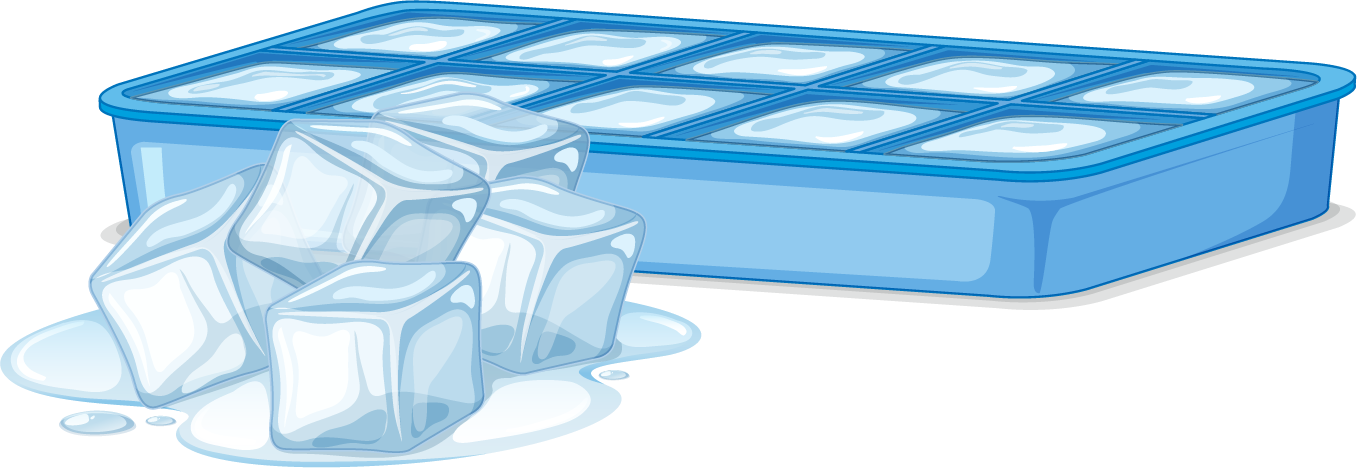Have you struggled to replace a new refill in an older pen?

This could have happened because the new refill is not the same size as the one you want to replace.

Remember that whenever identical objects are to be produced, the concept of congruence is taken into consideration in making the cast.

## What is Congruent?

### Congruent: Definition

The word "congruent" means equal in every aspect or figure in terms of shape and size.

Congruence is the term used to describe the relation of two figures that are congruent.

Let us do a small activity.

Draw two circles of the same radius and place one on another.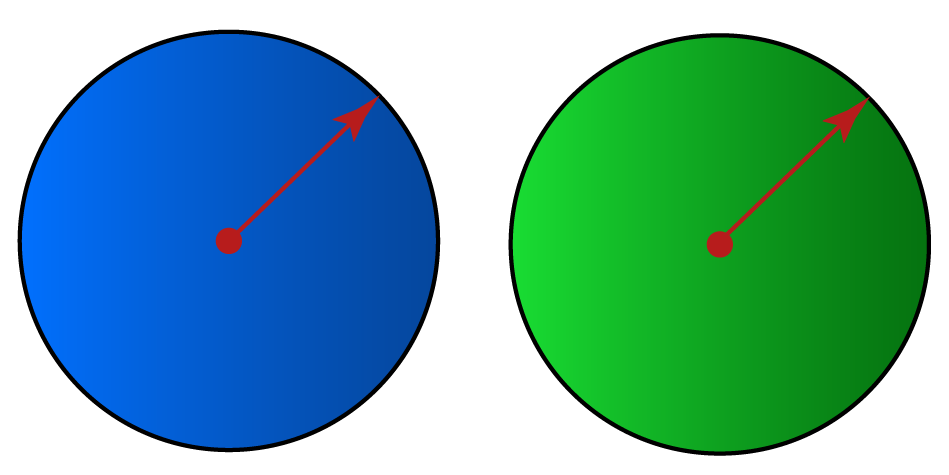Do they cover each other completely?

Yes, they do.

Hence, we can say that they are congruent circles.

Use the following simulation to explore more congruent shapes.

## Congruent Triangles

Now let's discuss congruence of two triangles.

Look at $$\Delta ABC$$ and $$\Delta PQR$$ below.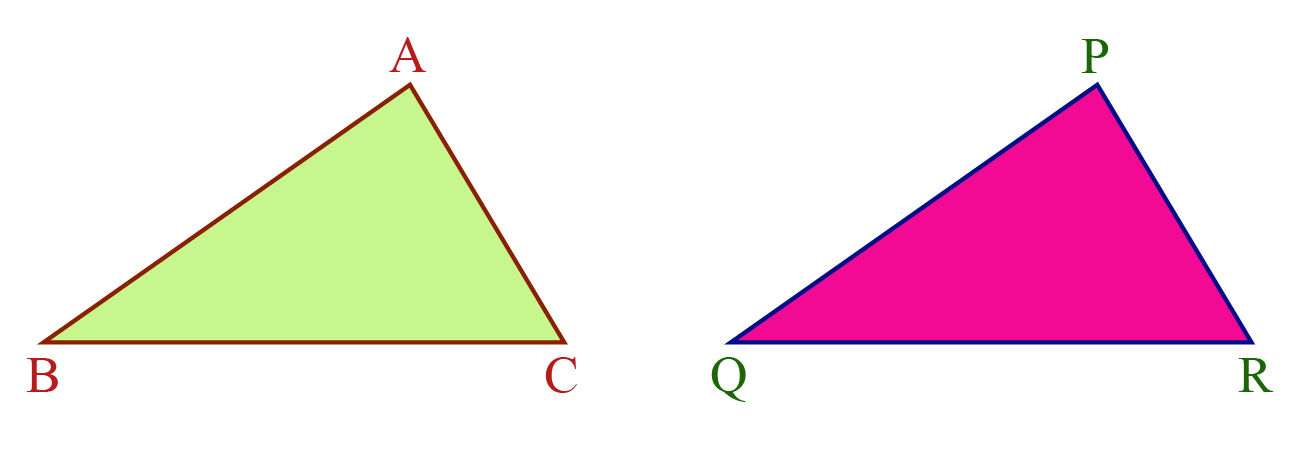These two triangles are of the same size and shape.

Thus, we can say that they are congruent.

We can represent this in a mathematical form using the congruent symbol.

$$\Delta ABC \cong \Delta PQR$$

This means $$A$$ falls on $$P$$, $$B$$ falls on $$Q$$ and $$C$$ falls on $$R$$.

Also, $$AB$$ falls on $$PQ$$, $$BC$$ falls on $$QR$$ and $$AC$$ falls on $$PR$$.

This indicates that the corresponding parts of congruent triangles are equal.

Congruent Parts of $$\mathbf{\Delta ABC}$$ and $$\mathbf{\Delta PQR}$$
Corresponding Vertices

$$A$$ and $$P$$

$$B$$ and $$Q$$

$$C$$ and $$R$$

Corresponding Sides

$$\overline{AB}$$ and $$\overline{PQ}$$

$$\overline{BC}$$ and $$\overline{QR}$$

$$\overline{AC}$$ and $$\overline{PR}$$

Corresponding Angles

$$\angle A$$ and $$\angle P$$

$$\angle B$$ and $$\angle Q$$

$$\angle C$$ and $$\angle R$$

Remember that it is incorrect to write $$\Delta BAC \cong \Delta PQR$$ because $$A$$ corresponds to $$P$$, $$B$$ corresponds to $$Q$$ and $$C$$ corresponds to $$R$$.

## Properties of Congruent Triangles

### Property 1

SSS Criterion for Congruence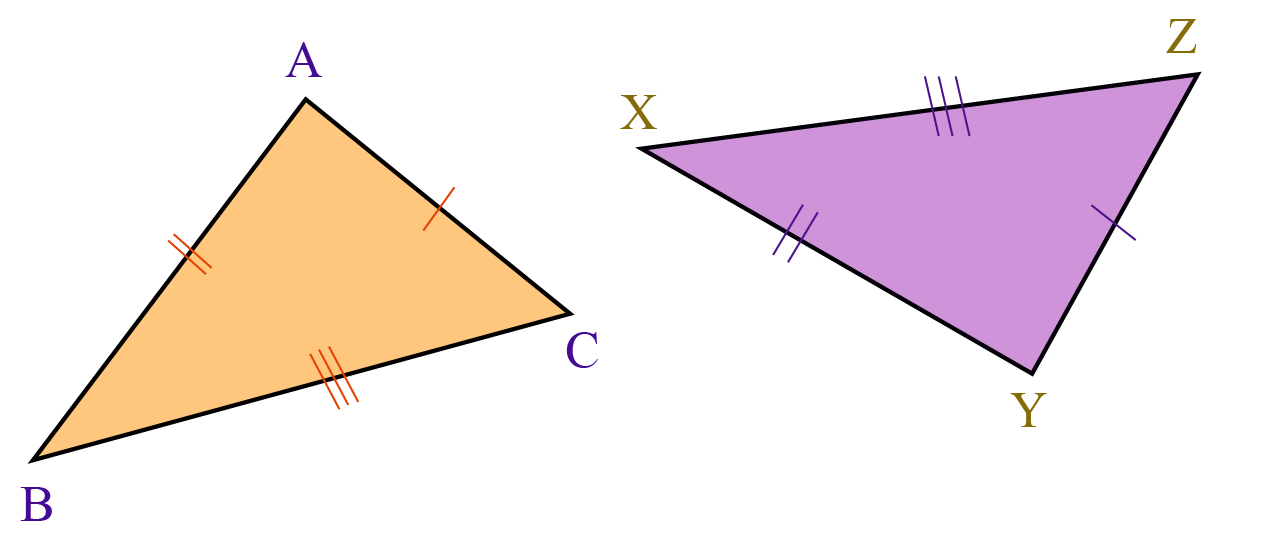SSS Criterion stands for Side-Side-Side Criterion.

Under this criterion, if the three sides of one triangle are equal to the three corresponding sides of another triangle, the two triangles are congruent.

### Property 2

SAS Criterion for Congruence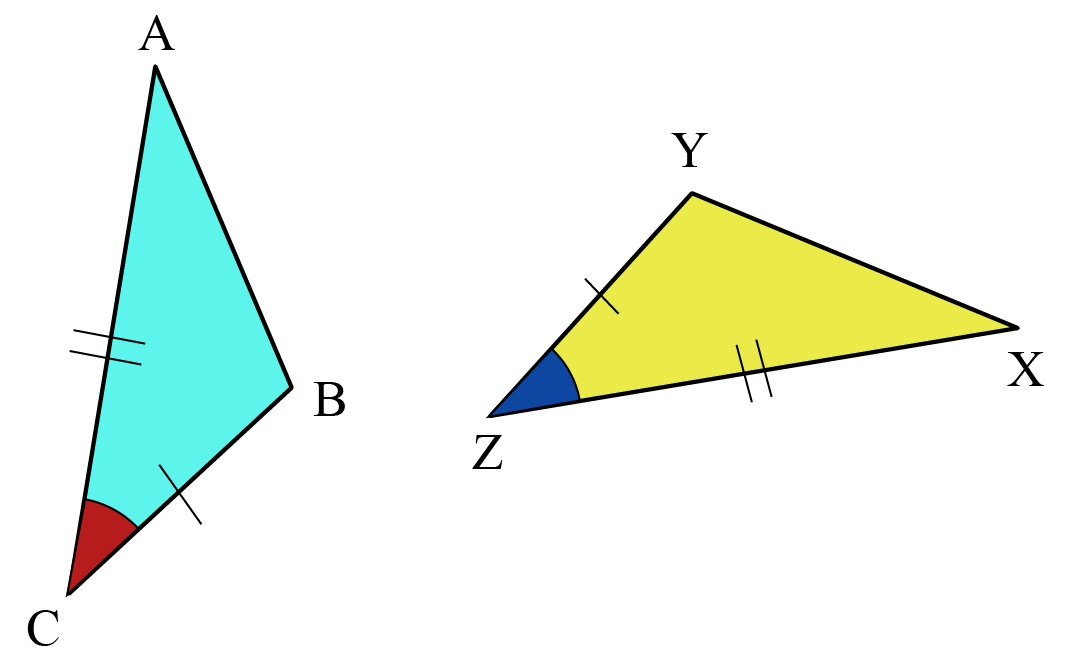SAS Criterion stands for Side-Angle-Side Criterion.

Under this criterion, if the two sides and the angle between the sides of one triangle are equal to the two corresponding sides and the angle between the sides of another triangle, the two triangles are congruent.

### Property 3

ASA Criterion for Congruence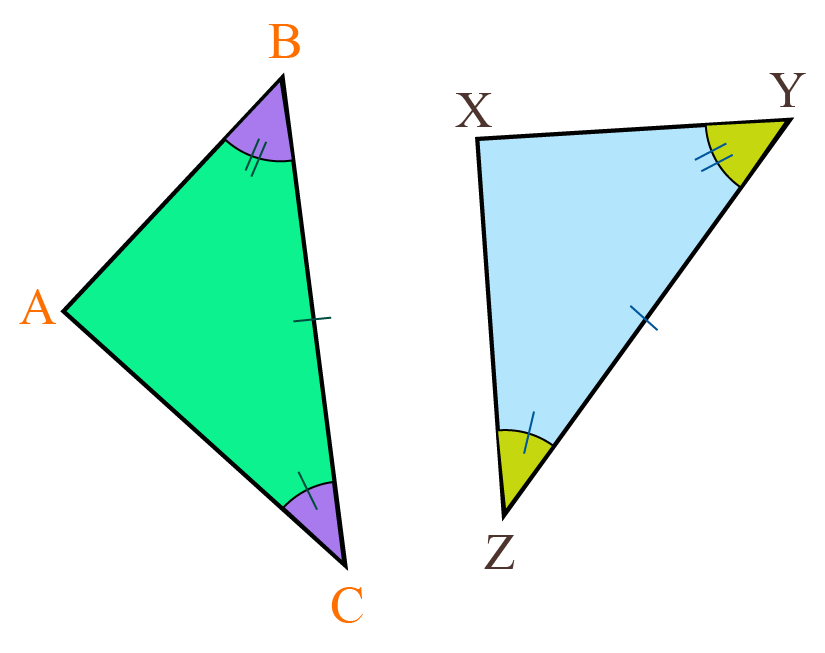ASA Criterion stands for Angle-Side-Angle Criterion.

Under this criterion, if the two angles and the side included between them of one triangle are equal to the two corresponding angles and the side included between them of another triangle, the two triangles are congruent.

### Property 4

AAS Criterion for Congruence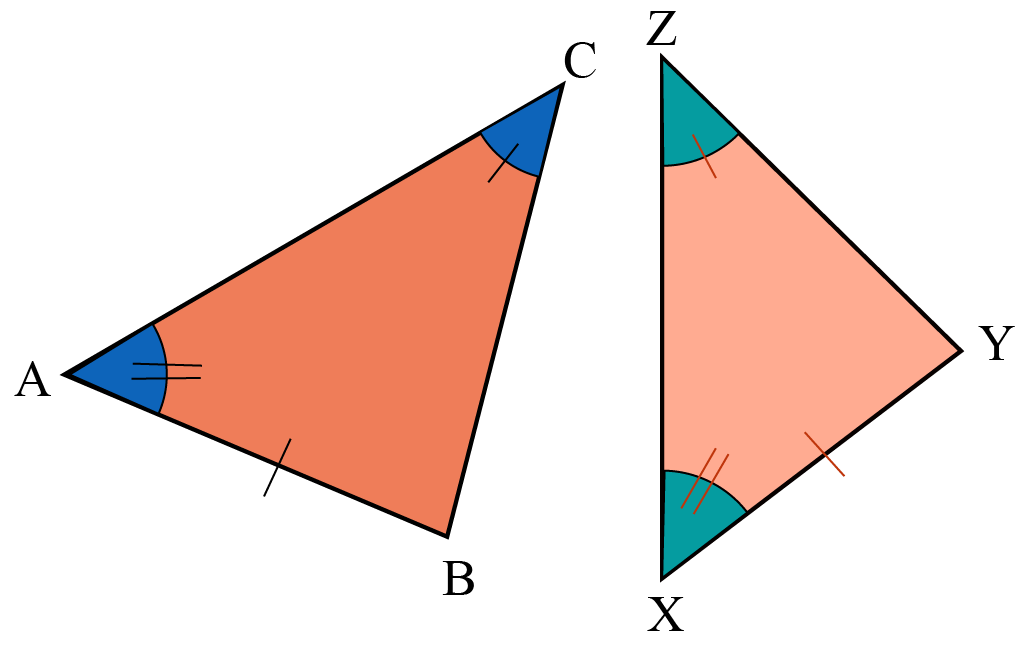AAS Criterion stands for Angle-Angle-Side Criterion.

Under this criterion, if the two angles and the non-included side of one triangle are equal to the two corresponding angles and the non-included side of another triangle, the triangles are congruent.

### Property 5

RHS Criterion for Congruence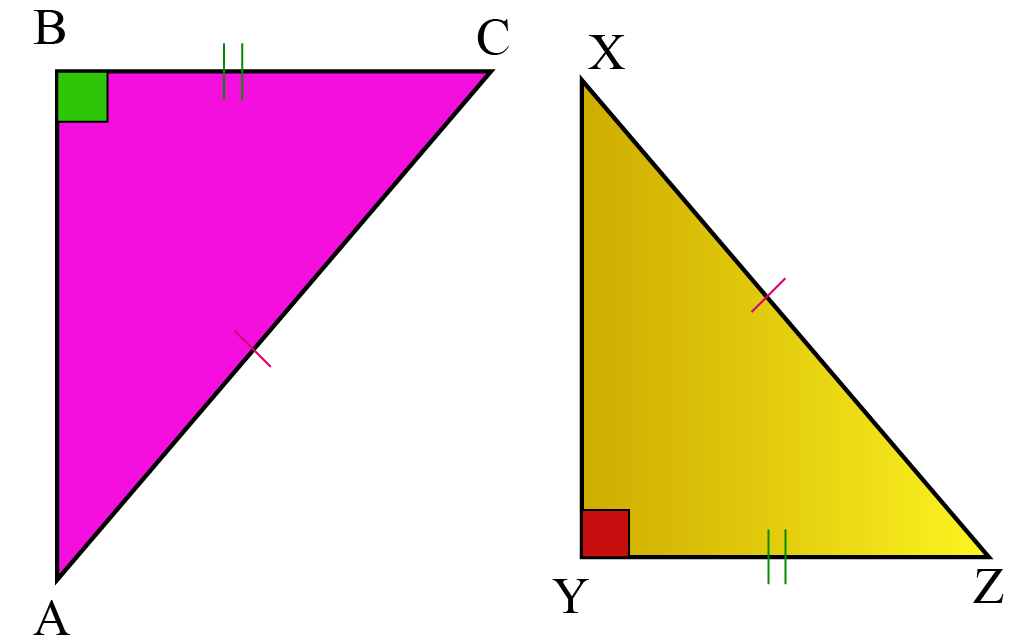RHS Criterion stands for Right Angle-Hypotenuse-Side Criterion.

Under this criterion, if the hypotenuse and side of one right-angled triangle are equal to the hypotenuse and the corresponding side of another right-angled triangle, the two triangles are congruent.

Explore these properties of congruent using the simulation below.

Download Triangles and Quadrilaterals Worksheets
Triangles and Quadrilaterals
grade 9 | Questions Set 1
Triangles and Quadrilaterals
grade 9 | Answers Set 1
Triangles and Quadrilaterals
grade 9 | Questions Set 2
Triangles and Quadrilaterals
grade 9 | Answers Set 2

More Important Topics
Numbers
Algebra
Geometry
Measurement
Money
Data
Trigonometry
Calculus